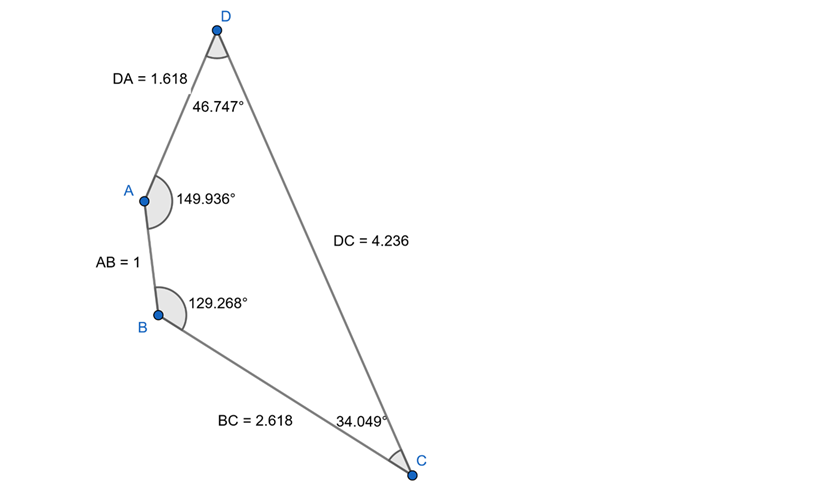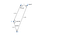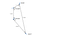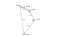More Golden Quadrilaterals, This Time Based on Sides

By Jim FonsecaFigure 1: A Golden Lateral Quadrilateral with Side of 1 Opposite 4.236 (Perimeter = 9.472; sum of angles = 360.000)

My previous posts on Medium introduced a new type of Golden Triangle based on its three angles in the proportion of the Golden Ratio. Then I showed how the same approach could be used to define Golden Quadrilaterals with their four angles in proportion to the Golden Ratio: 160.997°, 99.502°, 61.495° and 38.006°.

You can see the full research article and references posted on ResearchGate

https://www.researchgate.net/publication/350326954_Introducing_A_New_Golden_Triangle_Golden_Quadrilaterals_a_Golden_Irregular_Pentagon_and_Other_Phigons

But what about sides?

We can create what I will call Golden Lateral Quadrilaterals — four-sided figures with sides in Φ proportion. (I know that the phrase lateral quadrilateral is redundant but it’s helpful to distinguish these figures from the Golden Angular Quadrilaterals in my prior post.) Figure 1 above, and Figures 2 and 3 below, show the three possible arrangements of opposing sides for this type of quadrilateral.Figure 2: A Golden Lateral Quadrilateral with Side of 1 Opposite 1.618 (Perimeter = 9.472; sum of angles = 360.000)Figure 3: A Golden Lateral Quadrilateral with Side of 1 Opposite 2.618 (Perimeter = 9.472; sum of angles = 360.000)

Keep in mind that the angles shown in all three figures are illustrative, not fixed. That is because, while the lengths of sides and the perimeters of these quadrilaterals are fixed, the sides of the figures can be moved about their four corners, thus changing the angles, the shape and the area of each figure. Think of it this way: if you made these figures out of sticks with pivots in the corners, you could slide the sides around and change the shape of the figures.

I did not detect any Φ relationships in the angles of these three figures, but undoubtedly, just as it was possible to build two sides in Φ proportion into the phiangular quadrilateral, it is likely that we can build some angles in Φ proportion into these philateral quadrilaterals (probably two, maybe three?), although I have not attempted to do so. I’ll leave some of the fun to others. Michael De Villiers has also done research on Golden Quadrilaterals, a Golden Rhombus and a Golden Parallelogram with two sides in Φ proportion.

Do we have to stop at four sides? No. Can we create A Golden Irregular Pentagon with sides in Φ proportion? Yes, as shown below in Figure 4.Figure 4: A Golden Irregular Pentagon (Perimeter = 16.326; sum of angles = 539.99)

As with the philateral quadrilaterals, the angles of the irregular pentagon in Figure 4 are illustrative, not fixed, because the sides can move about the corners. In addition many other arrangements of the sides of the irregular pentagon are possible; the figure does not have to be constructed with the sides in sequential Φ sequence as in the figure above. Any of these changes would change the angles and the area of the figure but not the perimeter. Again, I did not detect any Φ relationships in the angles that happen to be shown in Figure 12, but undoubtedly, it is likely that we can build some angles in Φ proportion into these figures, although I did not attempt to do so.

I also assume it is possible to construct additional philateral polygons, such as a philateral hexagon, although I have not done so. Perhaps there is no limit to the theoretical number of polygons that can be constructed, but, as a practical matter, illustrating such multisided figures quickly gets out of hand. The longest side becomes impossible to portray in any meaningful way. For example, if it is possible to construct a philateral decagon, the longest side would be more than 100 times the length of the smallest side.

Terminology

Earlier in this paper you saw that I proposed the terms Golden Right Triangle, Golden Angle Quadrilaterals, Golden Lateral Quadrilaterals and Golden Irregular Pentagon for various figures.

More generally, I propose calling any type of phi-related polygon, including the traditional figures such as the Golden Gnomon, the Golden Triangle and the Kepler Triangle, phiangular polygons and philateral polygons, as appropriate.

Collectively we could call all these types of figures phigons. (This term should not be confused with the musical group or the science fiction character of the same name, lol.)

For those who want a refresher about the derivation of the Golden Ratio, I included that in my previous two posts on Medium.com and in my research article on ResearchGate. Fun stuff!

## More from Jim Fonseca

Retired as Dean and geography professor at Ohio University Zanesville. I spend time in Florida, Maine, Chicago and Newport, Rhode Island.